# Obtuse angle

Here is everything you need to know about an obtuse angle, including what it is and how to identify it.

Students first learn about obtuse angles in 4 th grade with their work in geometric measurements. They expand that knowledge as they progress through middle school.

## What is an obtuse angle?

An obtuse angle is an angle greater than 90^{\circ} but less than 180^{\circ} .

All obtuse angles fall
somewhere in between
these two angles.

Obtuse angles can be formed when two rays extend from a common point.

For example,

The symbol (\angle) is used to name an angle. The angle can be named after the vertex and a point on each ray or just the vertex.

The obtuse angle above can be named \angle \mathrm{F}, \angle \mathrm{YFT} or \angle \mathrm{TFY} .

If you picture a \, 90^{\circ} angle (shown in blue), it is clear that \angle \mathrm{YFT} \, is greater than \, 90^{\circ} , but less than 180^{\circ} (a straight line).

Visualizing a \, 90^{\circ} angle lets you identify most obtuse angles, but when in doubt, measure the angle with a protractor to prove that an angle is obtuse.

Obtuse angles are also formed by the sides of 2D shapes (polygons).

For example,

This octagon has all obtuse interior angles.

Some shapes have some obtuse angles, but also other types of angles.

For example,

Both the parallelogram and the trapezoid have two obtuse angles, but also other angles.

Obtuse angles are also formed when two straight lines cross and they are not perpendicular.

For example,

Two obtuse angles are formed when these lines cross, but there are also other angles.

### What is an obtuse angle?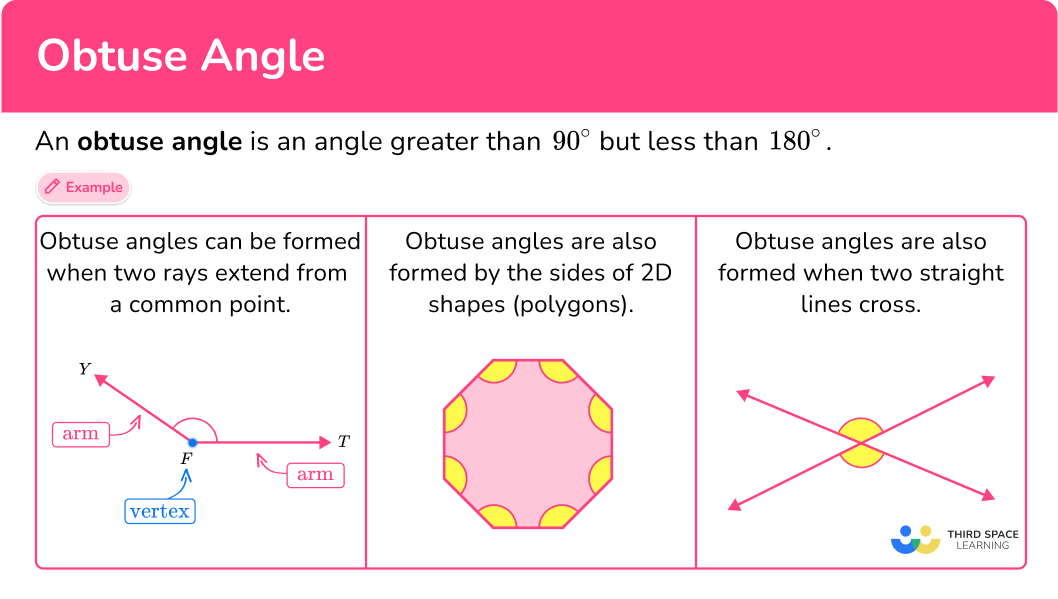## Common Core State Standards

How does this relate to 4 th grade math?

• Grade 4 – Measurement and Data (4.MD.C.5.a)
An angle is measured with reference to a circle with its center at the common endpoint of the rays, by considering the fraction of the circular arc between the points where the two rays intersect the circle. An angle that turns through \, \cfrac{1}{360} \, of a circle is called a “one-degree angle,” and can be used to measure angles.

• Grade 4 – Measurement and Data (4.MD.C.5.b)
An angle that turns through n one-degree angles is said to have an angle measure of n degrees.

• Grade 4 – Geometry (4.G.A.1)
Draw points, lines, line segments, rays, angles (right, acute, obtuse), and perpendicular and parallel lines. Identify these in two-dimensional figures.

## How to identify an obtuse angle

In order to identify an obtuse angle:

1. Recall the definition of an obtuse angle.
2. Explain whether or not the angle is obtuse.

## Obtuse angle examples

### Example 1: classify a given angle

Is the angle an obtuse angle?

1. Recall the definition of an obtuse angle.

An obtuse angle is an angle greater than 90^{\circ} but less than 180^{\circ} .

2Explain whether or not the angle is obtuse.

Comparing the angle to a 90^{\circ} angle (in blue), the angle is NOT an obtuse angle, because it is less than 90^{\circ} .

### Example 2: classify a given angle

Is the angle an obtuse angle?

An obtuse angle is an angle greater than 90^{\circ} but less than 180^{\circ} .

Comparing the angle to a \, 90^{\circ} angle (in blue), the angle is an obtuse angle, because it is greater than 90^{\circ} but less than 180^{\circ} .

### Example 3: identify obtuse angles in a given shape

How many obtuse angles does this regular pentagon have?

An obtuse angle is an angle greater than 90^{\circ} but less than 180^{\circ} .

Comparing the angle to a \, 90^{\circ} angle (in blue), every angle is an obtuse angle, because they are greater than 90^{\circ} but less than 180^{\circ} .

This shape (regular pentagon) has 5 obtuse angles.

### Example 4: identify obtuse angles in a given shape

How many obtuse angles does this rectangle have?

An obtuse angle is an angle greater than 90^{\circ} but less than 180^{\circ} .

Comparing the angle to a \, 90^{\circ} angle (in blue), all the angles are NOT obtuse angles, because they are equal to 90^{\circ} .

A rectangle has no obtuse angles.

### Example 5: identify obtuse angles in intersecting lines

How many obtuse angles are formed by these crossing lines?

An obtuse angle is an angle greater than 90^{\circ} but less than 180^{\circ} .

There are four angles formed by the crossing lines. The top and bottom angles are clearly greater than 90^{\circ} but less than 180^{\circ} .

The left and right angles are clearly less than 90^{\circ} .

There are 2 obtuse angles formed by these crossing lines.

### Example 6: identify real-life examples of obtuse angles

How many obtuse angles do you see in the scissors?

An obtuse angle is an angle greater than 90^{\circ} but less than 180^{\circ} .

There are two angles formed by the scissors. They are clearly greater than 90^{\circ} but less than 180^{\circ} .

There are 2 obtuse angles in the scissors.

### Teaching tips for an obtuse angle

• Provide students with plenty of opportunities to practice drawing obtuse angles or identifying them in real life. Always encourage students to justify their classification of an obtuse angle by comparing it to a \, 90^{\circ} angle (this can be the corner of a piece of paper) or measuring it with a protractor.

• Instead of using just worksheets, have students practice creating obtuse angles by using a clock (either a physical math manipulative or a digital version). Students can practice moving the minute hand and hour hand to make obtuse angle measures. As a bonus, they can also practice telling the time they have created.

### Easy mistakes to make

• Confusing obtuse angles with other types of angles
There are different types of angles besides obtuse: acute angles are between 0^{\circ} and \, 90^{\circ} , right angles are \, 90^{\circ} , straight angles are 180^{\circ} , reflex angles are between 180^{\circ} and 360^{\circ} , and full angles are 360^{\circ} . Without repeated practice and exposure, it is easy to get the vocabulary words confused.

• Thinking the angles of a triangle can’t be obtuse
Many triangles do not have an obtuse angle, such as acute triangles and right triangles. However, a triangle can have an obtuse angle. Obtuse triangles (obtuse angle triangles) have two acute angles and one obtuse angle.
For example,

• Making incorrect assumptions about angles close to \, \bf{90^{\circ}} or \bf{180^{\circ}}
It can be hard to tell if angles that measure around 89^{\circ} or 189^{\circ} are obtuse or not. When in doubt, always measure with a protractor.
For example,
The angles below are too close to tell and need to be measured with a protractor to verify the type of angle.

### Obtuse angle practice questions

1) Is the angle obtuse? Why or why not?Yes, because it is less than 90^{\circ}No, because it is less than 90^{\circ}Yes, because it is greater than 90^{\circ} and less than 180^{\circ}No, because it is greater than 90^{\circ} and less than 180^{\circ}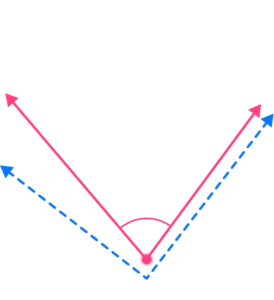Comparing the angle to a 90^{\circ} angle (in blue), the angle is NOT an obtuse angle, because it is less than 90^{\circ} .

2) Is the angle obtuse? Why or why not?Yes, because it is greater than 90^{\circ} and less than 180^{\circ}No, because it is greater than 90^{\circ} and less than 180^{\circ}Yes, because it is less than 90^{\circ}No, because it is less than 90^{\circ}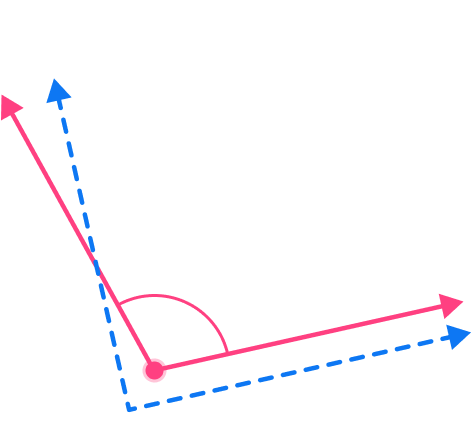Comparing the angle to a 90^{\circ} angle (in blue), the angle is an obtuse angle, because it is greater than 90^{\circ} and less than 180^{\circ} .

3) How many obtuse angles does the hexagon have?2345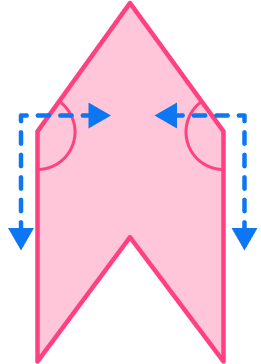Comparing the angle to a \, 90^{\circ} angle (in blue), these two angles are obtuse angles, because they are greater than 90^{\circ} and less than 180^{\circ} .These three angles are NOT obtuse angles, because they are less than 90^{\circ} .This angle is NOT an obtuse angle, because it is greater than 180^{\circ} .

This irregular hexagon has 2 obtuse angles.

4) Which shape has 1 obtuse angle?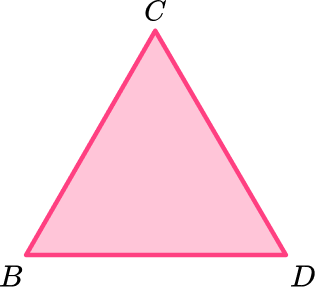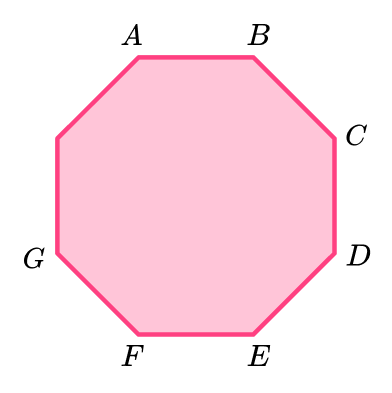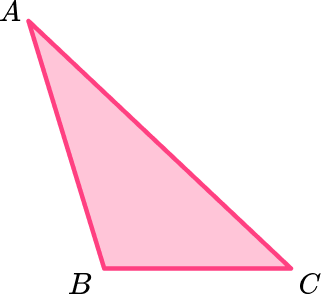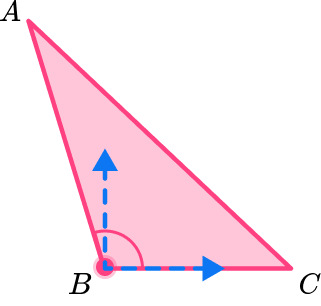Comparing the angle to a \, 90^{\circ} angle (in blue), triangle ABC has one obtuse angle, because it is greater than 90^{\circ} and less than 180^{\circ} .

The other two angles in the triangle are acute angles.

5) Which angles are obtuse?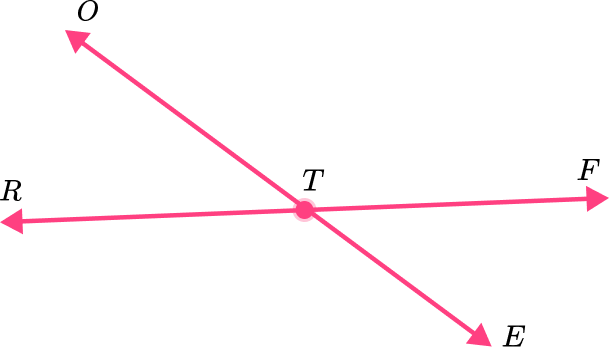\angle R T E \, and \angle E T F\angle \mathrm{ETF} \, and \angle \mathrm{FTO}\angle R T E \, and \angle \mathrm{FTO}\angle E T F \, and \angle R T O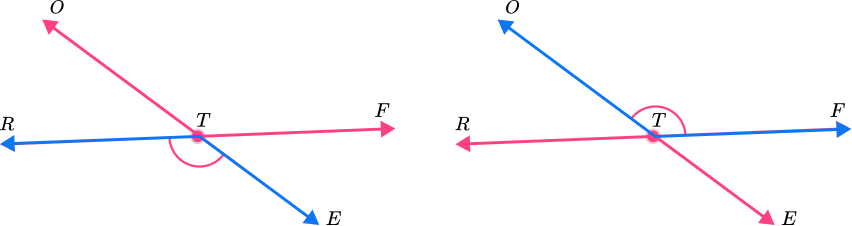These two angles (\angle R T E and \angle \mathrm{FTO}) are greater than 90^{\circ} and less than 180^{\circ} , so they are obtuse angles.

6) How many obtuse angles does the clock have?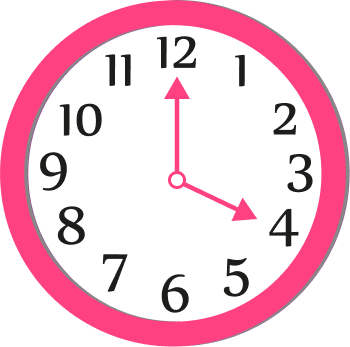0123There are two angles formed by the arms of the clock. The angle shown is clearly greater than 90^{\circ} and less than 180^{\circ} .

There is one obtuse angle in the clock.

## Obtuse angle FAQs

Can an isosceles triangle have an obtuse angle?

Yes, an isosceles triangle has 2 acute angles, but the third angle can be acute, right or obtuse.

Can an equilateral triangle have an obtuse angle?

No, because an equilateral triangle has three congruent 60 degree angles, none of the angles are obtuse.

Can a right triangle have an obtuse angle?

No, because a right triangle has two acute angles and one 90 -degree angle, none of the angles are obtuse.

When classifying by angles, which types of triangles have obtuse angles?

Only obtuse triangles have an obtuse angle. Acute triangles and right triangles do not have an obtuse angle.

## Still stuck?

At Third Space Learning, we specialize in helping teachers and school leaders to provide personalized math support for more of their students through high-quality, online one-on-one math tutoring delivered by subject experts.

Each week, our tutors support thousands of students who are at risk of not meeting their grade-level expectations, and help accelerate their progress and boost their confidence.